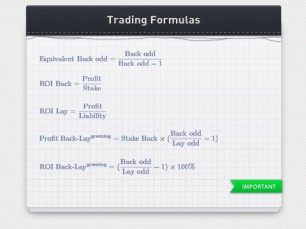This site uses cookies. When you browse the site you are consenting to its use. Know more.

## Useful formulas for Sports Betting TradingThere are some useful formulas than a trader should know, if for no other reason, to demystify a little the Sports Betting Trading world. There is no secret to winning money with odd variations and it´s not a dark science.

In trading jargon we say that a trade is a pair of opposite "bets" in the same selection of a market. That is, to bet for (Back) an event and then to bet against (Lay), or vice versa.

On Betfair, the biggest betting exchange in the world, we can start a trade by buying a bet and then win money whether the market rises or falls.

Buying a bet is to lay (to bet against) and selling a bet is to Back (to bet in favour).

We win money with a trade if we back at a higher odd than the lay odd – for the same selection of the same market:

• If the market is rising we must first Lay and then Back;
• If the market is falling we must first Back and then Lay.

• 1. Formula to calculate how much to bet to close a trade
• 2. Formula to calculate the liability of a Lay bet
• 3. Formula to convert Lay odd in Back odd
• 4. Formula to calculate the profitability of a bet
• 5. Formula to calculate the profitability of a trade

## 1.Formula to calculate how much to bet to close a tradeThat is, if our trade started with a Back:By closing a trade with a stake calculated by “How much to bet”, we are dividing the profit evenly throughout all selections, that is, we are Hedging.

Almost all trading software (like Betangel or GeeksToy) have this function incorporated to close a trade by automatically dividing profit/loss throughout all selections of the market with a single mouse click.

Online, there are also many calculators available which will allow you to make such a calculation.

## 2.Formula to calculate the liability of a Lay bet

When we Lay at a given stake, if the odd is lower than 2 we are risking less money than the value of the stake, but if the odd is higher than 2 we are risking more money than the stake. The amount of money we risk is called liability.## 3.Formula to convert Lay odd in Back odd

In any given market, there are two possible outcomes: an event will occur or not.

Therefore, the probability of an event happening plus the probability of not happening must always be 100%.

If we add that to the fact that probability is the inverse of the odd, we can convert the Lay odd in a equivalent Back odd that represents that same event:Example:

The probability of the home team winning a football match is 75%, therefore, the odd to bet in favour of the home team (back odd) is:Accordingly, the odd of the home team not winning that match is 25%, so by betting against the home team (home team Lay) it's as if we were Backing at an odd of:Usually, it is easier to understand if we are risking a lot for a small price when we look at odds of bets in favour (Back odds).

Actually, at Betfair we would be Laying with an odd of 1.33 (to match someone's Back), but the value of our Lay stake is not the amount of money we are risking. The value of our Lay is the "Lay stake" and the amount of money we risk is the "liability", as we saw above.

Therefore, it can be easier, at least in the beginning, when we don't have much trading practise, to convert our Lay odd in an "equivalent Back odd" to have a better understanding of the bet we are placing. A Lay @1.33 is equivalent to a Back @4.00.

## 4.Formula to calculate the profitability of a bet

The concept of profitability is expressed in percentage of the amount of money risked.

If we place a Back bet (to bet in favour):If we place a Lay bet (to bet against):## 5.Formula to calculate the profitability of a trade

In generic terms, the profitability of a trade is the profit of the trade (or pair of bets) divided by the amount of money risked on the first bet.

If we first Back and then Lay:

» In case the profit is not distributed and is left in the selection where we made the trade:» In case the profit is distributed amongst all selections of the market:If we first Lay and then Back:

» In case the profit is not distributed and is left in the selection where we made the trade:» In case the profit is distributed amongst all selections of the market:Did sports betting trading become any easier?
I hope so!

Share "Useful formulas for Sports Betting Trading" via: